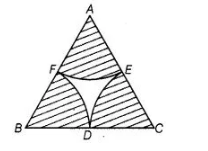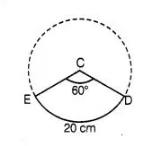# In figure arcs are drawn by taking vertices A, B

Question:

In figure arcs are drawn by taking vertices A, B and C of an equilateral triangle of side 10 cm, To intersect the sides BC, CA and AB at their respective

mid-points D, E and F. Find the area of the shaded region, (use π = 3.14)Solution:

Since, ABC is an equilateral triangle.

$\angle A=\angle B=\angle C=60^{\circ}$

and $\quad A B=B C=A C=10 \mathrm{~cm}$

So, $E, F$ and $D$ are mid-points of the sides.$\therefore \quad A E=E C=C D=B D=B F=F A=5 \mathrm{~cm}$

Now, area of sector $C D E=\frac{\theta \pi r^{2}}{360}=\frac{60 \times 3.14}{360}(5)^{2}$

$=\frac{3.14 \times 25}{6}=\frac{78.5}{6}=13.0833 \mathrm{~cm}^{2}$

$\therefore \quad$ Area of shaded region $=3$ (Area of sector $C D E$ )

$=3 \times 13.0833$

$=39.25 \mathrm{~cm}^{2}$